• ## matlab函数拟合

千次阅读 2017-02-19 22:31:00
1 函数拟合 函数拟合在工程（如采样校正）和数据分析（如隶属...仅对常用的多项式拟合和已知函数类型的拟合中部分matlab函数的使用进行介绍。 1.1多项式拟合 对于 形式的拟合函数，其中 为待定系数。我们可以使...

1 函数拟合
函数拟合在工程（如采样校正）和数据分析（如隶属函数确定）中都是非常有用的工具。我这里将函数拟合分为三类:分别是多项式拟合，已知函数类型的拟合和未知函数类型的拟合。matlab中关于函数的拟合提供了很多的拟合函数，这里不再一一介绍。仅对常用的多项式拟合和已知函数类型的拟合中一部分matlab函数的使用进行介绍。
1.1多项式拟合
对于 形式的拟合函数，其中 为待定系数。我们可以使用matlab中的polyfit函数进行拟合。函数的调用形式为：
coef = polyfit(xx,yy,n);
其中xx,yy分别为已知的自变量和因变量数据，n为拟合的阶次。下面是一个使用的例子。
clear
clc   %清除

%设置参数
xx = [1,2,3,4,5];
yy = [6.1,7.2,8.1,9.2,10.1];
n = 1;     %选择一阶拟合

%下面代码不用修改
coef = polyfit(xx,yy,n);
%上面已经求出结果，下面是进行绘图显示
Fun = poly2sym(coef)    %显示拟合函数
xmax = max(xx);
xmin = min(xx);
xnum = 2*length(x1)+50;
x = linspace(xmin,xmax,xnum);
y = polyval(coef,x);
plot(xx,yy,'o',x,y);
title(char(Fun))
运行完成后，会在matlab命令行窗口显示Fun=x+257/50.同时会绘图如下：上面这段代码可以直接拿来使用，只需要改动xx,yy,n即可。后面的代码不需要修改。为了进一步方便使用，我简单的制作了一个GUI界面，同样只需要设定相关值，点击开始拟合就可以拟合了。简单的GUI界面 如下所示：1.2已知函数类型的拟合
我们在数据分析的时候经常遇到这种情况：知道了函数的隶属函数和隶属函数上的一些点，求隶属函数的待定系数。由于隶属函数基本都不是多项式的形式，于是我们就不能使用ployfit函数了。这里我以拟合偏大型柯西分布隶属函数和对数隶属函数为例，介绍fit和nlinfit在已知函数类型时的拟合应用。 关于函数的具体用法我这里就不介绍了，大家可以在matlab中doc一下帮助文件，也可以上网查询相关介绍。如果着急使用又不想了解原理的话可以直接修改下面程序中需要拟合的数据和函数类型即可。 假设需要拟合的偏大型柯西分布隶属函数和对数函数组合的分段函数如下所示： 其中待定系数 同时给出已知数据点 分别采用fit和nlinfit对第二段偏大型柯西分布隶属函数拟合代码如下：
clear
clc   %清除工作空间
syms x;

%公共参数设置
xx=[3,5]';   %这里设置已知自变量向量(列向量)
yy = [0.8,1]';  %对应因变量(列向量)
startPos = [1,1];   %设置系数的起始搜索点
%使用fit函数拟合的
%设置参数
f = '(1+alpha*(x-belta)^(-2))^(-1)';  %设置需要拟合的函数形式
funType=fittype(f,'independent','x',...
'coefficients',{'alpha','belta'});  %在independent后面设置自变量,在coefficients后面设置待定系数（多个值用{}括起来）
%使用nlinfit函数进行拟合的
%设置参数
f1 = @(coef,x)(1+coef(1)*(x-coef(2)).^(-2)).^(-1);  %设置需要拟合的函数(内联函数形式)

%后面的代码不用改
%fit拟合相关代码
opt=fitoptions(funType);
set(opt,'StartPoint',startPos);
cfun=fit(xx,yy,funType,opt)   %命令行显示结果
plot(cfun,'r',xx,yy,'*')

%nlinfit拟合相关代码
coef=nlinfit(xx,yy,f1,startPos);
disp('nlinfit拟合后的系数矩阵为：');
disp(coef);

hold on
xmax = max(xx);
xmin = min(xx);
xnum = 2*length(xx)+50;
x = linspace(xmin,xmax,xnum);
y = f1(coef,x);
plot(x,y,'g');

legend('原始数据','fit拟合','nlinfit拟合')
两种方法的拟合结果会在命令行中显示，同时给出拟合绘图结果如下图所示：函数使用介绍：
只需要在参数设置的地方设置相关参数即可。nlinfit函数拟合时采用内联函数的形式，需要主要系数和自变量都是一个向量，自己要将向量的每个元素和实际系数对应好。fit拟合函数采用符号表达式的形式，所以函数看起来就顺眼多了。但是那些符号是自变量哪些是系数要写清楚。事实上，这两个函数不仅支持单自变量拟合，还可以进行多自变量拟合，但是后面不需要改的代码也要自己该。因为plot绘制不了多变量图形。
函数特点介绍：
一般这种函数的拟合都采用的搜索算法，因此得到的解基本没用“最优解”（如果有最优解的话使用solve应该能解出来）。搜索算法都比较依赖第一个初始搜索点，startPos的设置也非常关键。不同的起始搜索点得到的结果可能不一样。当然搜索算法的不同得到的结果也可能不一样（比如上面两种）。这也是matlab中有那么多拟合函数的原因(还有lsqcurvefit,regress等)。将每个函数看出一个专家的话，这个诊断不出来，可以换一个专家看看。 照猫画虎我们可以对前面的对数函数进行拟合。修改设置参数部分代码如下：
%公共参数设置
xx=[1,3]';   %这里设置已知自变量向量(列向量)
yy = [0.01,0.8]';  %对应因变量(列向量)
startPos = [1,1];   %设置系数的起始搜索点
%使用fit函数拟合的
%设置参数
f = 'a*log(x)+b';  %设置需要拟合的函数形式
funType=fittype(f,'independent','x',...
'coefficients',{'a','b'});  %在independent后面设置自变量,在coefficients后面设置待定系数（多个值用{}括起来）
%使用nlinfit函数进行拟合的
%设置参数
f1 = @(coef,x)coef(1)*log(x)+coef(2);  %设置需要拟合的函数(内联函数形式)
同样命令空间会给出拟合后的结果以及显示拟合后的曲线。可以看出这回两种方法拟合的结果完全一样，后面绘制的曲线已经完全挡住了先绘制的。采用不同的方法，不同的起始搜索位置得到了相同的结果往往说明这个结果非常接近全局最优点。
ps:关于函数形式未知的函数拟合，我好久都没用到过了也懒得总结。或许以后再用到时会将这一部分内容补上吧！或许吧~~其实如果我们不知道函数的形式，使用插值往往更好一些。因为函数的拟合往往都需要运气，并不一定能成功。而插值基本都会成功了。

转载于:https://www.cnblogs.com/yabin/p/6417566.html
展开全文• MATLAB“fitgmdist”函数拟合高斯混合模型(维数据)在MATLAB中“fitgmdist”的用法及其GMM聚类算法中介绍过"fitgmdist"函数的用法，这次"fitgmdist"拟合一维数据。1. 维高斯混合数据的产生function data=...

MATLAB用“fitgmdist”函数拟合高斯混合模型(一维数据)
在MATLAB中“fitgmdist”的用法及其GMM聚类算法中介绍过"fitgmdist"函数的用法，这次用"fitgmdist"拟合一维数据。
1. 一维高斯混合数据的产生
function data=generate_GMM()
%前两列是数据，最后一列是类标签
%数据规模
N=300;
%数据维度
% dim=1;
%%
%混合比例
para_pi=[0.3 0.2 0.5];
%第一类数据
mul=0; % 均值
S1=1; % 协方差
data1=mvnrnd(mul, S1, para_pi(1)*N); % 产生高斯分布数据
%第二类数据
mu2=4;
S2=2;
data2=mvnrnd(mu2,S2,para_pi(2)*N);
%第三类数据
mu3=-4;
S3=1;
data3=mvnrnd(mu3,S3,para_pi(3)*N);
data = [data1, ones(para_pi(1)*N,1); data2, 2*ones(para_pi(2)*N,1); data3, 3*ones(para_pi(3)*N,1)];
save data_gauss data
2. 拟合高斯混合模型
data=data(:,1);
K=3;
gmm=fitgmdist(data, K);
% 自定义参数
% RegularizationValue=0.001; %正则化系数，协方差矩阵求逆
% MaxIter=1000; %最大迭代次数
% TolFun=1e-8; %终止条件
% gmm=fitgmdist(data, K, 'RegularizationValue', RegularizationValue, 'CovarianceType', 'diagonal', 'Start', 'plus', 'Options', statset('Display', 'final', 'MaxIter', MaxIter, 'TolFun', TolFun));
[N, D]=size(data);
mu=gmm.mu; %均值
Sigma=gmm.Sigma; %协方差矩阵
ComponentProportion=gmm.ComponentProportion; %混合比例
Y=zeros(N, K);
for k=1:K
Y(:,k)=ComponentProportion(k).*normpdf(data, mu(k), Sigma(:,:,k));
end
YY=sum(Y, 2);
plot(data,YY,'r.')
3. 结果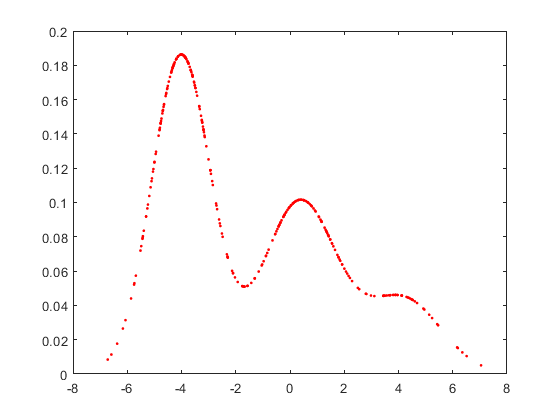4. 参考

展开全文• X=xlsread(‘E:\DATA\data1.xlsx’,‘sheet1’,‘B2:B13’);  ...%函数拟合如下 Xsum=0; Ysum=0; mol=0; den=0; for i=1:length(X) Xsum=Xsum+X(i); Ysum=Ysum+Y(i); end Xmean=Xsum/12; Ymean=Y
X=xlsread(‘E:\DATA\data1.xlsx’,‘sheet1’,‘B2:B13’);   %读取X数据 Y=xlsread(‘E:\DATA\data1.xlsx’,‘sheet1’,‘C2:C13’);   %读取Y数据
%函数拟合如下 Xsum=0; Ysum=0; mol=0; den=0; for i=1:length(X) Xsum=Xsum+X(i); Ysum=Ysum+Y(i); end Xmean=Xsum/12; Ymean=Ysum/12; for i=1:length(X) mol=mol+(X(i)-Xmean)(Y(i)-Ymean); den=den+(X(i)-Xmean)^2; end b=mol/den; a=Ymean-bXmean;   %求出a，b值，即可得到拟合函数方程y=a+bx;
%显著性F检验如下 Yfore=[]; for i=1:length(X) Yfore(i)=-0.65*X(i)+3.85;  %-0.65为上面求出的b值，3.85为上面求出的a值 end Q=0; U=0; for i=1:length(X) Q=Q+(Y(i)-Yfore(i))^2; U=U+(Yfore-Ymean)^2; end F=U/(Q/(12-2));   %查F表进行显著性检验
想了解更多，请关注微信公众号“GAO戏精光芒万丈”：展开全文• 本文主要记录常用的维插值与函数拟合使用方法 文章目录前言维插值二、函数拟合2.1 多项式拟合2.1.1多项式拟合指令2.1.2 图形窗口的多项式拟合2.2 指定函数拟合参考资料 前言 最近在学习数学建模，这部分...
本文主要记录Matlab中常用的一维插值与函数拟合的使用方法

文章目录
前言一、一维插值二、函数拟合2.1 多项式拟合2.1.1多项式拟合指令2.1.2 图形窗口的多项式拟合
2.2 指定函数拟合
参考资料

前言
最近在学习数学建模，这部分关于怎么使用Matlab，刚好记录下来，做电赛的时候这些常用的插值、拟合工具也是需要学习的，刚开始学，写的不好，欢迎大家批评指正。

以下是本篇文章正文内容
一、一维插值
用MATLAB软件求解插值问题  在MATLAB中提供了一个一维插值函数interp1， 它的调用格式为 cy=interp1(x , y , cx , ‘method’) 其中x、y是所给数据的横纵坐标，要求x的分量按升序或降序排列，cx是待求的插值点的横坐标，返回值cy是待求的插值点的纵坐标，method是插值方法， 该函数提供了四种可选的插值方法：
nearest——最邻近点插值。 点和这两已知点间位置的远近来进行插值，取较近已知 它根据已知两点间的插值 插值点处的函数值作为未知插值点处的函数值。linear——线性插值。它将相邻的数据点用直线相连， 按所生成的直线进行插值。spline——三次样条插值。它利用已知数据求出样条 函数后，按样条函数进行插值。cubic——三次插值。它利用已知数据求出三次多项式 函数后，按三次多项式函数进行插值。（在我的Matlab2019b中名为pchip） 缺省时插值方法为分段线性插值。
下面用该函数来求解下列插值问题。 对于下面给定的4组数据，求在x=110处 y的值。
xy10010121111441216913
很明显，我们可以看出这组数据符合函数y=sqrt(x); 我们用科学计算器得到一个解cy*=10.4880。 接下来我们使用Matlab进行插值计算： 输入命令：
x=[100 121 144 169];
y=[10 11 12 13];
cx=110;
cy1=interp1(x,y,cx,'nearest');
cy2=interp1(x,y,cx,'linear');
cy3=interp1(x,y,cx,'pchip');
cy4=interp1(x,y,cx,'spline');

运行结果依次为 cy1 = 10、cy2 =10.4762、cy3 = 10.4869、cy4 = 10.4877 通过比较cy* = 10.4880，显然三次样条插值的结果最好。
二、函数拟合
2.1 多项式拟合
将数据点按多项式的形式进行拟合，使用最小二乘法可以确定多项式的系数，多项式拟合有指令语句和图形窗口两种方法。
2.1.1多项式拟合指令
polyfit(X,Y,N):多项式拟合，返回降幂排列的多项式系数。 polyval(P,xi):计算多项式的值。 其中，X，Y是数据点的值；N是拟合的最高次幂；P是返回的多项式系数；xi是要求点的横坐标。 例如对下面的数据进行多项式拟合。
x123456789y9763-125720
拟合的命令如下：
x = [1 2 3 4 5 6 7 8 9];
y = [9 7 6 3 -1 2 5 7 20];
P = polyfit(x,y,3);
xi = 0:0.2:10;
yi = polyval(P,xi);
plot(xi,yi,x,y,'r*');

得到的拟合曲线与原始数据的对照如图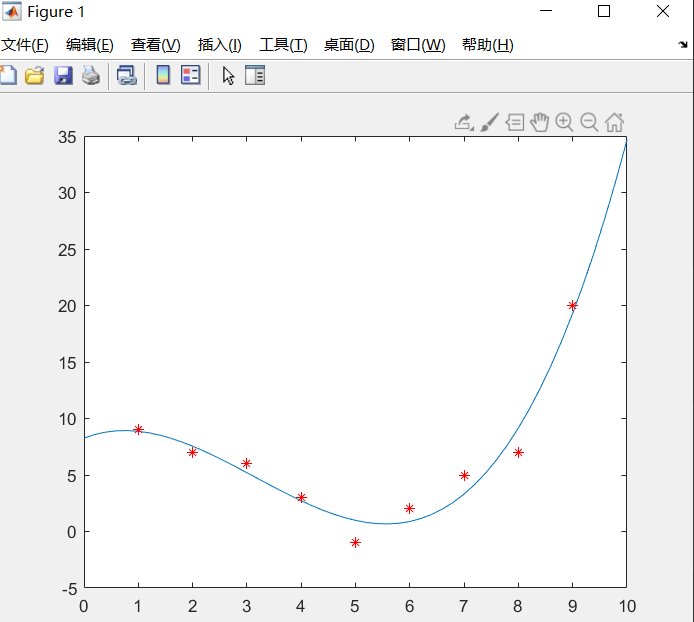2.1.2 图形窗口的多项式拟合
在图形窗口可以用菜单方式对数据进行简单、快速、搞笑的拟合。与2.1.1数据相同，先画出数据点：
x = [1 2 3 4 5 6 7 8 9];
y = [9 7 6 3 -1 2 5 7 20];
plot(x,y,'r*');

然后在图形窗口单击Tools(工具)->Basic Fitting(基本拟合)，打开对话框，并按下图进行操作，其中分别用线性、二阶、三阶对数据进行了多项式拟合，下面的柱状图显示残差，可以看出，进行三阶多项式拟合的效果是最好的，与前述结果一样。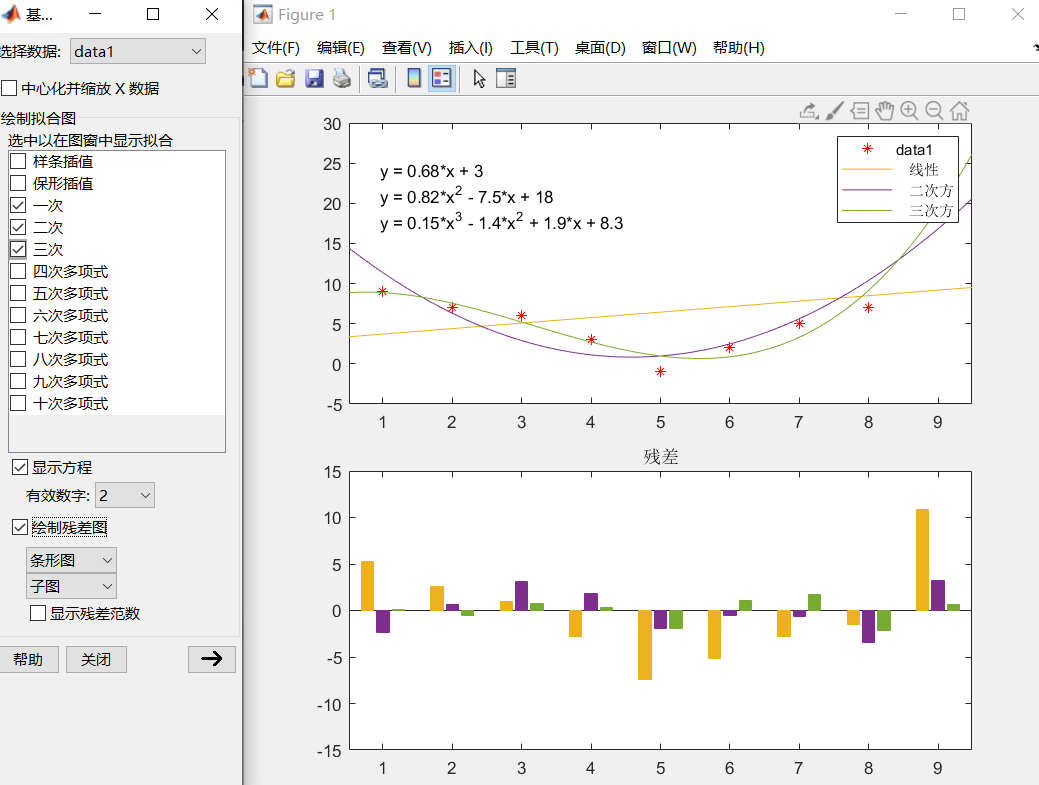2.2 指定函数拟合
在Matlab中也可以用用户自定义的函数进行拟合。通过下面的例子可以了解用指定函数进行数据拟合的基本方法。
x00.41.222.83.64.45.267.289.210.411.612.413.614.415y10.850.29-0.27-0.53-0.4-0.120.170.280.15-0.03-0.15-0.0710.0590.080.032-0.015-0.02
在Matlab中先用命令画出上述的散点图：
x = [0 0.4 1.2 2 2.8 3.6 4.4 5.2 6 7.2 8 9.2 10.4 11.6 12.4 13.6 14.4 15];
y = [1 0.85 0.29 -0.27 -0.53 -0.4 -0.12 0.17 0.28 0.15 -0.03 -0.15 -0.071 0.059 0.08 0.032 -0.015 -0.02];
plot(x,y,'r*');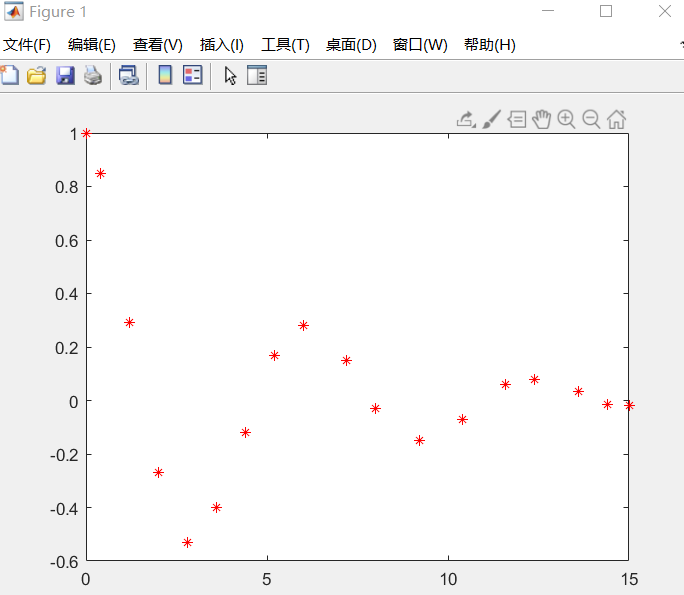知道其对应的函数形式为

f

(

t

)

=

a

c

o

s

(

k

t

)

e

w

t

f(t) = acos(kt)e^{wt}

,则可用Matlab进行拟合，程序如下：
syms t
x = [0;0.4;1.2;2;2.8;3.6;4.4;5.2;6;7.2;8;9.2;10.4;11.6;12.4;13.6;14.4;15];
y = [1;0.85;0.29;-0.27;-0.53;-0.4;-0.12;0.17;0.28;0.15;-0.03;-0.15;-0.071;0.059;0.08;0.032;-0.015;-0.02];
f = fittype('a*cos(k*t)*exp(w*t)','independent','t','coefficients',{'a','k','w'});
cfun = fit(x,y,f);
xi = 0:0.1:20;
yi = cfun(xi);
plot(x,y,'r*',xi,yi,'b-');

运行结果如图：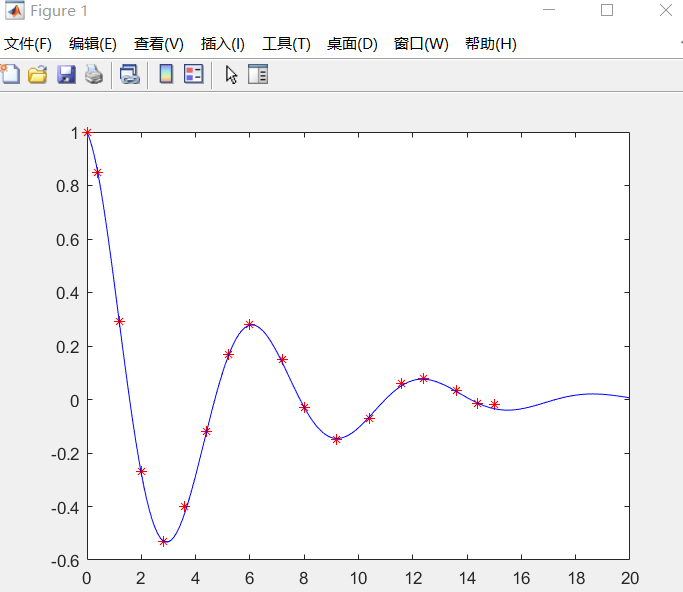参考资料
《MATLAB在数学建模中的应用》卓金武《北京航空航天大学出版社》 , 2011.4

有用的话点个赞吧！
展开全文matlab 数学建模
• import numpy as npimport matplotlib.pyplot as pltfrom scipy.optimize import curve_fit#python拟合函数最主要模块就是cure_fit#准备数据x=[一组数据]y=[一组数据]#定义你自己想要拟合函数def func(x,E0,B0,...
• 　最近应该是六叔的物化理论作业要交了吧，很多人问我六叔的作业里面有两道题要怎么进行图像函数拟合。综合起来的问题主要有两个： 　1. 知道图像的准确拟合方程，但是不知道怎么拟合。(这个是本文的重点) 　2. ...
• 提出种基于传感器温度补偿的双指数函数拟合算法，方面采用双指数函数对非线性的温度系数曲线进行补偿，另方面在双指数函数拟合的算法中，提出种具有高精度初值的交替迭代法。该方法首先利用四数据点计算出...传感器
• import numpy as npimport matplotlib.pyplot as pltfrom scipy.optimize import curve_fit#python拟合函数最主要模块就是cure_fit#准备数据x=[一组数据]y=[一组数据]#定义你自己想要拟合函数def func(x,E0,B0,...
• 然后程序尝试使用 MatLab 函数“lsqcurvefit”拟合数据，以找到二维高斯的位置、方向和宽度。 不输入参数执行“mainD2GaussFitRot.m”。 二维高斯函数函数“D2GaussFunctionRot.m”和“D2GaussFunction.m”定义matlab
• ## matlab 函数拟合polyfit

万次阅读 多人点赞 2017-09-12 20:16:38
1、matlab做线性最小二乘拟合 函数命令为：a=polyfit(x,y,m) % x，y为对应的自变量，m为需要拟合的最高次幂  y=polyval(a,x); %根据拟合函数得出x对应的因变量的值 ...例如：给出一组数据,拟合变化过程如
• 不论是 matlab 还是 R 语言，根据一组数据拟合分布函数时，只能拟合出特定分布的参数值，并不能给出最适合这组数据的分布函数。例如，matlab 有 normfit 函数来计算一组数据拟合成正态分布时的均值和方差，wblfit ...
• 给定了一组[x,y]，让我们去拟合一个指数函数，要如何做？ 不卖关子： 设待拟合函数为 y=k∗xay=k*x^{a}y=k∗xa 需要求出kkk和aaa. 思路是两边取对数，将指数函数拟合问题转换成线性函数 变换后： log(y)=log(k...算法
• 多项式函数拟合：a=polyfit(xdata,ydata,n) 其中n表示多项式的最高阶数，xdata，ydata为将要拟合的数据，它是数组的方式输入．输出参数a为拟合多项式 的系数  多项式在x处的值y可用下面程序计算．  y=...matlab
• 代价函数参数引入(监督学习)代价函数单个参数优化拟合过程(θ0 = 0的情况下)两个参数情况下的优化过程(θ1与θ0都参与优化) 参数引入(监督学习) Hypothesis是假设函数，假设该函数的两个参数:y = θ1x + θ0,θ1是...机器学习
• 在Python中使用神经网络算法拟合函数使用梯度下降算法优化模型参数。提供了详细的代码，并详细解释了实现过程。可以帮助读者更好地理解神经网络算法如何拟合函数神经网络 梯度下降法 最优化 机器学习
• 个简单实例，介绍在TensorFlow中以双层人工神经网络进行函数拟合的过程。TensorFlow 机器学习 神经网络
• 个简单实例，介绍在TensorFlow中以单层人工神经网络进行函数拟合的过程。TensorFlow 机器学习 神经网络
• 最近在看李航的统计学习方法P11时发现个多项式函数拟合问题觉得公式的推导有问题，于是看了一些资料发现这里的推倒是有错误的，python编程验证后发现按书上的求导结果拟合后的函数图像完全不对，下面给出正确的...统计学习方法 机器学习 python
• 推导过程：  1. 设拟合多项式为：    2. 各点到这条曲线的距离之和，即偏差平方和如下：    3. 为了求得符合条件的a值，对等式右边求ai偏导数，因而我们得到了：
• 多项式拟合： x=1:10; y=randn(size(x)); p=polyfit(x,y,7); % 7阶多项式，返回拟合多项式的降幂系数 ...或者直接使用matlab的拟合工具箱 写好x, y值 x=1:10; y=randn(size(x)); 打开工具箱 或者在命令行输入...
• ## 拟合函数

千次阅读 2017-10-12 15:44:40
• 拟合拟合问题的目标是寻求函数(曲线)，使得该曲线在某种准则下与所有的数据点最为接近，即曲线拟合的最好(最小化损失函数)。必须有函数表达式、不一定通过所有数据点。二、模型适用范围或案例功能：...
• 其中一种常见的形式为：二次函数拟合。 方法 获取实验数据x, y 利用np.polyfit(x, y, 2)进行二次拟合 得到拟合出的系数，进行后续的数据处理 实例 已知一组二次曲线型数据，要求拟合出该曲线，并且返回最大点/对称...python
• 本工具箱用于时间序列的时间函数拟合预测，适用于：已知一组时间序列，并且有明显的趋势，要求预测未来时间的数据。 本工具箱基于 多元线性回归模型，将非线性函数转化为线性函数，可通过此工具箱打开线性回归工具...
• 其中b为拟合函数的斜率k值 import numpy as np import matplotlib.pyplot as plt x=np.array([12,54,62,84,69,33,50]) y=np.array([14,15,67,32,45,56,21]) xx,xy=0,0 for i in range(len(x)): xx+=pow(x[i],2) ...python 算法
• 最小二乘法 最小二乘法： 已知四个数据点，寻找一条直线（曲线），使得每个点与线的...已知一组数据点，满足函数y=ax+b，利用最小二乘法的原理求解a和b。 图中数字10是有10个数据点 matlab代码 %% 线性拟合 Linear fmatlab 线性代数 算法
• 一组三次样条拟合到给定的数据，并返回一个内联函数matlab...